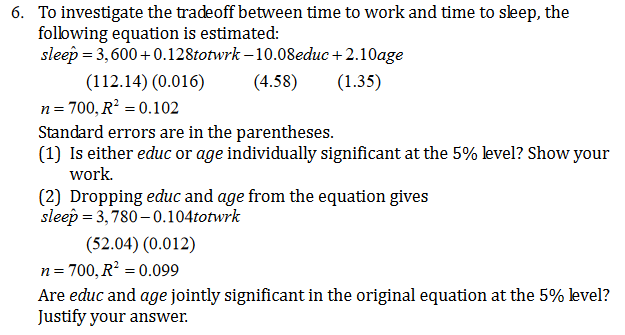# To investigate the tradeoff between time to work and time to sleep, thefollowing equation is estimated:sleep 3,600+0.128totwrk -10.08educ+2.10age6.(112.14) (0.016)n 700, R2 0.102Standard errors are in the parentheses(1) Is either educ or age individually significant at the 5% level? Show yourwork(4.58)(1.35)(2) Dropping educ and age from the equation givessleep 3,780-0.104totwrk(52.04) (0.012)700, R2 0.099nAre educ and age jointly significant in the original equation at the 5% level?Justify your answer

Question
242 viewshelp_outlineImage TranscriptioncloseTo investigate the tradeoff between time to work and time to sleep, the following equation is estimated: sleep 3,600+0.128totwrk -10.08educ+2.10age 6. (112.14) (0.016) n 700, R2 0.102 Standard errors are in the parentheses (1) Is either educ or age individually significant at the 5% level? Show your work (4.58) (1.35) (2) Dropping educ and age from the equation gives sleep 3,780-0.104totwrk (52.04) (0.012) 700, R2 0.099 n Are educ and age jointly significant in the original equation at the 5% level? Justify your answer fullscreen
check_circle

Step 1

6

1.

Testing for independent variable “educ”:

State the null and alternative Hypotheses:

Null Hypothesis:

H0: The variable “educ” is not significant.

Alternative Hypothesis:

H1: The variable “educ” is significant.

From the given information, the coefficient of  “educ” is -10.08 and the corresponding standard error is 4.58. The sample size is 700.

The test statistic is 2.2009 and it is calculated below:

Step 2

The number of sample observations is n=700 and consider the level of significance as 0.05.

The degree of freedom is, – 1 = 700–1 = 699.

Here, the tail of the test is two tail test.

The p-value is 0.0281 and it is by Excel function “=T.DIST.2T(2.2009,699)”.

Rejection Rule:

If p-value ≤ α, then reject the null hypothesis.

If p-value > α, then do not reject the null hypothesis.

Conclusion:

The p-value is 0.0281 and the level of significance is 0.05.

Here, the p-value is lesser than the level of significance.

From the rejection rule,  reject the null hypothesis.

That is, the variable “educ” is significant.

Step 3

Testing for independent variable “age”:

State the null and alternative Hypotheses:

Null Hypothesis:

H0: The variable “age” is not significant.

Alternative Hypothesis:

H1: The variable “age” is significant.

From the given information, the coefficient of  ...

### Want to see the full answer?

See Solution

#### Want to see this answer and more?

Solutions are written by subject experts who are available 24/7. Questions are typically answered within 1 hour.*

See Solution
*Response times may vary by subject and question.
Tagged in

### Other